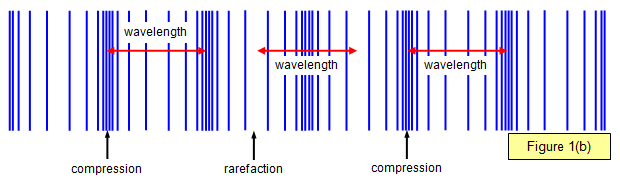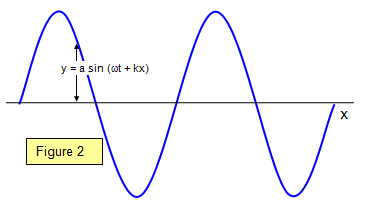Wave motion

A wave motion is the transmission of energy from one place to another through a material or a vacuum. Wave motion may occur in many forms such as water waves, sound waves, radio waves and light waves, but the waves are basically of only two types:

transverse waves - the oscillation is at right angles to the direction of propagation of the wave (Figure 1(a)). Examples of this type are water waves and most electromagnetic waves.(b) longitudinal waves - the oscillation is along the direction of propagation of the wave (Figure 1(b)). An example of this type is sound waves.Basic definitions:

 Wavelength: the distance between any two successive corresponding points on the wave, for example that between two maxima or two minima (λ) Displacement: the distance from the mean, central, undisturbed (y) Amplitude: the maximum displacement (a) Frequency: the number of vibrations per second made by the wave (f) Period: the time taken for one complete oscillation (T= 1/f) Phase: a term related to the displacement at zero time (ε)
(see 16-19/Wave properties/Wave properties/Text/Phase change)

Basic Wave equation

The velocity, frequency and wavelength of a wave are given by the formula:

Wave velocity (v) = frequency of waves (f) x wavelength of waves (λ)

Wave equationsWe will consider here the motion of a sine wave (Figure 2), since this type is the most fundamental. However it can be shown that any other wave may be built up from a series of sine waves of differing frequency.

We can express a wave travelling in the positive x direction by the equation:

Positive x direction :     y = a sin(ωt – kx)

and for one travelling in the opposite direction:

Negative x direction :     y = a sin(ωt + kx)

where k is a constant and w = 2pf.

The sign gives the direction of the motion. We can separate each equation into two terms:
(a) a term showing the variation of displacement with time at a particular place - for example, when x = 0 y = a sin (ωt), that is, the variation of displacement with time at the particular place x = 0.
(b) a term showing the variation of displacement with distance at a particular time - for example, when t = 0 y = a sin (kx), that is, the variation of displacement with distance at a particular time t = 0.

An alternative form of the equation can be proved as follows.

Since the period T = 1/f where f is the frequency and ω = 2πf we have ω = 2π/T.
Also when t = 0 y = 0 at x = 0, λ/2, λ...and so on, and so k = 2πλ. The equation may therefore be written:

y = a sin 2π(t/T + x/λ)

Example problem
A certain travelling wave has frequency (f) of 200 Hz, a wavelength (λ) of 2m and an amplitude (a) of 0.02 m.
Calculate the displacement (y) at a point 0.3m from the origin at a time 0.01s after zero displacement at that point.

The period of the wave = 1/f = 1/200 = 0.005 s-1

y = a sin 2π(t/T + x/λ) = 0.02 sin[2π(0.01/0.005 + 0.3/2)]
= 0.02 sin[2π(2 + 0.15)] = 0.02 sin[13.5] = 0.02x0.81 = 0.016 m

A VERSION IN WORD IS AVAILABLE ON THE SCHOOLPHYSICS USB